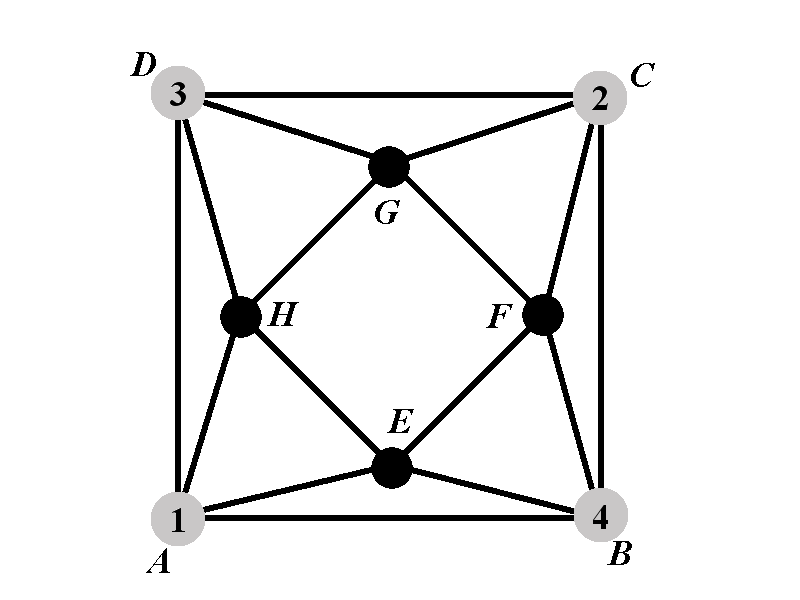Back to: Chromatic Polynomial
##### Case 2.2: Calculation of $$P_{2.2^{\circ}}(k)$$We will now consider two sub-cases: Sub-case $$2.2.1^{\circ}$$ is when the vertex $$H$$ is of one of the colors $$\{2,4\}$$; and sub-case $$2.2.2^{\circ}$$ is when the vertex $$H$$ does not have one of the colors from $$\{2,4\}$$.

The number of proper colorings in which $$H$$ has color $$2$$ is equal to the number of proper colorings in which $$H$$ is of color $$4$$. Therefore we will cacluate only the number of proper colorings in which $$H$$ has color $$2$$. We will denote by $$P_{2.2.1^{\circ}}(k)$$ the number of proper colorings in which $$H$$ has color $$2$$, and as specified earlier $$A$$, $$B$$, $$C$$, and $$D$$ have colors $$1$$, $$4$$, $$2$$, and $$3$$ respectively.

Notice that $$H$$ cannot have color $$1$$ nor color $$3$$. Therefore, if $$H$$ has a color outside of the set $$\{2,4\}$$, then $$H$$ must not have any of the colors from $$\{1,2,3,4\}$$. The number of colorings in the case $$2.2.2^{\circ}$$ is $$(k-4)\cdot P_{2.2.2^{\circ}}(k)$$ where $$P_{2.2.2^{\circ}}(k)$$ is the number of proper colorings of $$\Gamma$$ in which $$A$$, $$B$$, $$C$$, $$D$$, and $$H$$ have colors $$1$$, $$4$$, $$2$$, $$3$$, and $$5$$ respectively.

The number $$P_{2.2^{\circ}}(k)$$ satisfies $P_{2.2^{\circ}}(k)=2\cdot P_{2.2.1^{\circ}}(k)+(k-4)\cdot P_{2.2.2^{\circ}}(k).$

Therefore the polynomial $$P_{2.2^{\circ}}(k)$$ satisfies \begin{eqnarray*}P_{2.2^{\circ}}(k)&=&2\cdot P_{2.2.1^{\circ}}(k)+(k-4)\cdot P_{2.2.2^{\circ}}(k)\newline &=&2\left(k^3-9k^2+28k-31\right)+(k-4)\left(k^3-10k^2+36k-47\right)\newline &=&2k^3-18k^2+56k-62+(k-3)\left(k^3-10k^2+36k-47\right)-\left(k^3-10k^2+36k-47\right)\newline &=&k^3-8k^2+20k-15+(k-3)\left(k^3-10k^2+36k-47\right)\newline &=&k^3-3k^2-5k^2+15k+5k-15+(k-3)\left(k^3-10k^2+36k-47\right)\newline &=&(k-3)\left(k^2-5k+5\right)+(k-3)\left(k^3-10k^2+36k-47\right)\newline \ &=&(k-3)\left(k^3-9k^2+31k-42\right). \end{eqnarray*}